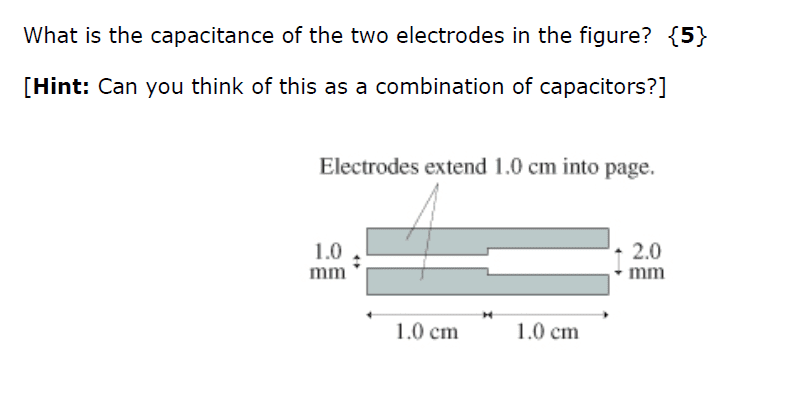# Capacitance of Two Electrodes

StillAnotherDave
Homework Statement:
Finding the capacitance of two electrodes...
Relevant Equations:
C=εA/dCan I get some help in answering this questions?

As I understand, for two electrodes:

C=εA/d

A = 0.012 m2
d = 0.001 m on the left and d = 0.002 m on the right.

Thus the capacitance of each part is:

Left: C = (εx0.012)/0.001

Right: C= (εx0.012)/0.002

Firstly, is this the right approach? Secondly, what next? Do you simply add the two values to get the overall capacitance?

Help greatly appreciated!

#### Attachments

Homework Helper
Gold Member
Firstly, is this the right approach?
YesSecondly, what next? Do you simply add the two values to get the overall capacitance?
Follow the hint. Can you consider these two capacitances as being in parallel or series? If so, which one?

StillAnotherDave
To me, they look to be in parallel. Hence C = C1 + C2. Correct?

Homework Helper
Gold Member
Yes. That's right.

StillAnotherDave
Great! Now if you can just turn your attention to my semi-infinite well question ...Appreciate the help.